The OpenFOAM Foundation
sensibleInternalEnergy< Thermo > Class Template Reference

Thermodynamics mapping class to expose the sensible internal energy functions. More...

## Public Member Functions

sensibleInternalEnergy ()
Construct. More...

scalar Cpv (const Thermo &thermo, const scalar p, const scalar T) const
Heat capacity at constant volume [J/(kg K)]. More...

scalar CpByCpv (const Thermo &thermo, const scalar p, const scalar T) const
Cp/Cv []. More...

scalar HE (const Thermo &thermo, const scalar p, const scalar T) const
Sensible internal energy [J/kg]. More...

scalar THE (const Thermo &thermo, const scalar e, const scalar p, const scalar T0) const
Temperature from sensible internal energy. More...

## Static Public Member Functions

static word typeName ()
Return the instantiated type name. More...

static word name ()

## Detailed Description

### template<class Thermo> class Foam::sensibleInternalEnergy< Thermo >

Thermodynamics mapping class to expose the sensible internal energy functions.

Definition at line 45 of file sensibleInternalEnergy.H.

## ◆ sensibleInternalEnergy()

 sensibleInternalEnergy ( )
inline

Construct.

Definition at line 53 of file sensibleInternalEnergy.H.

## ◆ typeName()

 static word typeName ( )
inlinestatic

Return the instantiated type name.

Definition at line 60 of file sensibleInternalEnergy.H.

## ◆ name()

 static word name ( )
inlinestatic

Definition at line 67 of file sensibleInternalEnergy.H.

References sensibleInternalEnergy< Thermo >::Cpv().

Here is the call graph for this function: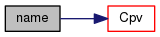## ◆ Cpv()

 scalar Cpv ( const Thermo & thermo, const scalar p, const scalar T ) const
inline

Heat capacity at constant volume [J/(kg K)].

Definition at line 74 of file sensibleInternalEnergy.H.

References sensibleInternalEnergy< Thermo >::CpByCpv().

Referenced by sensibleInternalEnergy< Thermo >::name().

Here is the call graph for this function: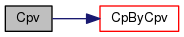Here is the caller graph for this function: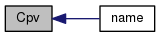## ◆ CpByCpv()

 scalar CpByCpv ( const Thermo & thermo, const scalar p, const scalar T ) const
inline

Cp/Cv [].

Definition at line 85 of file sensibleInternalEnergy.H.

References sensibleInternalEnergy< Thermo >::HE().

Referenced by sensibleInternalEnergy< Thermo >::Cpv().

Here is the call graph for this function: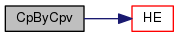Here is the caller graph for this function: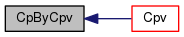## ◆ HE()

 scalar HE ( const Thermo & thermo, const scalar p, const scalar T ) const
inline

Sensible internal energy [J/kg].

Definition at line 96 of file sensibleInternalEnergy.H.

References sensibleInternalEnergy< Thermo >::THE().

Referenced by sensibleInternalEnergy< Thermo >::CpByCpv().

Here is the call graph for this function: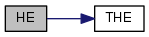Here is the caller graph for this function: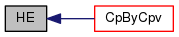## ◆ THE()

 scalar THE ( const Thermo & thermo, const scalar e, const scalar p, const scalar T0 ) const
inline

Temperature from sensible internal energy.

given an initial temperature T0

Definition at line 108 of file sensibleInternalEnergy.H.

Referenced by sensibleInternalEnergy< Thermo >::HE().

Here is the caller graph for this function: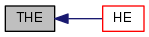The documentation for this class was generated from the following file: